# PyTorch基础学习总结

1、Tensor张量

2、Tensor数据类型

3、Tensor常用函数

1、简易神经网络模型

2、Pytorch自动梯度

3、使用自动梯度和自定义函数搭建简易神经网络模型

1、使用torch.nn搭建神经网络模型

2、使用torch.optim优化模型

# 一、PyTorch中的Tensor张量

## 1、Tensor张量

Tensor在PyTorch中负责存储基本数据，PyTorch针对Tensor也提供了相对丰富的函数和方法，所以PyTorch中的Tensor与NumPy的数组具有极高的相似性，同时 Tensor可以使用 GPU 进行计算。

## 2、Tensor数据类型

（1）torch.FloatTensor    # 此变量用于生成数据类型为浮点型的Tensor，传递给torch.FloatTensor的参数可以是一个列表，也可以是一个维度值；

import torch
a=torch.FloatTensor(2,3)   #生成数据类型为浮点型的Tensor
print(a)
b=torch.FloatTensor([2,3,4,5])
print(b)
tensor([[0., 0., 0.],
[0., 0., 0.]])
tensor([2., 3., 4., 5.])

（2）torch.IntTensor    # 用于生成数据类型为整型的Tensor

## 3、Tensor常用函数

（1）torch.rand    # 用于生成数据类型为浮点型且维度指定的随机Tensor，和在Numpy中使用numpy.rand生成随机数的方法类似，随机生成的浮点数据在0~1区间均匀分布。

（2）torch.randn    # 用于生成数据类型为浮点型且维度指定的随机Tensor，和在Numpy中使用numpy.randn生成随机数的方法类似，随机生成的浮点数的取值满足均值为0，方差为1的正太分布。

（3）torch.range    # 用于生成数据类型为浮点型且自定义其实范围和结束范围的Tensor，所以传递给torch.range的参数有三个，分别是范围的起始值，范围的结束值和步长，其中，步长用于指定从起始值到结束值的每步的数据间隔。

（4）torch.zeros    # 用于生成数据类型为浮点型且维度指定的Tensor，不过这个浮点型的Tensor中的元素值全部为0。

（5）torch.abs    # 将参数传递到torch.abs后返回输入参数的绝对值作为输出，输出参数必须是一个Tensor数据类型的变量。

（7）torch.clamp    # 对输入参数按照自定义的范围进行裁剪，最后将参数裁剪的结果作为输出。所以输入参数一共有三个，分别是需要进行裁剪的Tensor数据类型的变量、裁剪的上边界和裁剪的下边界，具体的裁剪过程是：使用变量中的每个元素分别和裁剪的上边界及裁剪的下边界的值进行比较，如果元素的值小于裁剪的下边界的值，该元素就被重写成裁剪的下边界的值；同理，如果元素的值大于裁剪的上边界的值，该元素就被重写成裁剪的上边界的值。

a=torch.randn(2,3)
print(a)
b=torch.clamp(a,-0.1,0.1)   #边界值裁剪
print(b)
tensor([[-0.6895, -0.2334, -1.1246],
[ 0.2961, -1.4742,  1.7083]])
tensor([[-0.1000, -0.1000, -0.1000],
[ 0.1000, -0.1000,  0.1000]])

（8）torch.div    # 将参数传递到torch.div后返回输入参数的求商结果作为输出，同样，参与运算的参数可以全部是Tensor数据类型的变量，也可以是Tensor数据类型的变量和标量的组合。

（9）torch.pow    # 将参数传递到torch.pow后返回输入参数的求幂结果作为输出，参与运算的参数可以全部是Tensor数据类型的变量，也可以是Tensor数据类型的变量和标量的组合。

（10）torch.mul    # 将参数传递到 torch.mul后返回输入参数求积的结果作为输出，参与运算的参数可以全部是Tensor数据类型的变量，也可以是Tensor数据类型的变量和标量的组合。

（11）torch.mm    # 将参数传递到 torch.mm后返回输入参数的求积结果作为输出，不过这个求积的方式和之前的torch.mul运算方式不太样，torch.mm运用矩阵之间的乘法规则进行计算，所以被传入的参数会被当作矩阵进行处理，参数的维度自然也要满足矩阵乘法的前提条件，即前一个矩阵的行数必须和后一个矩阵的列数相等，否则不能进行计算。

（12）torch.mv    # 将参数传递到torch.mv后返回输入参数的求积结果作为输出，torch.mv运用矩阵与向量之间的乘法规则进行计算，被传入的参数中的第1个参数代表矩阵，第2个参数代表向量，顺序不能颠倒。

（13）torch.view    # 改变一个 tensor 的大小或者形状。

x=torch.randn(4,4)
y=x.view(16)
z=x.view(-1,8)  #-1表示该维度大小是从其他维度推断出来的
print(x.size(),y.size(),z.size())
torch.Size([4, 4]) torch.Size() torch.Size([2, 8])

# 二、基于PyTorch搭建简易神经网络模型

## 1、简易神经网络模型

# coding:utf-8
import torch
batch_n = 100  #一个批次中输入数据的数量,值是100表示在一个批次中输入100个数据
input_data = 1000  #每个数据包含的数据特征
hidden_layer = 100 #经过隐藏层后保留的数据特征的个数
output_data = 10  #输出数据表示分类结果值的数量

#初始化权重
x = torch.randn(batch_n, input_data) #输入层维度为（100,1000）
y = torch.randn(batch_n, output_data) #输出层维度为（100,10）
w1 = torch.randn(input_data, hidden_layer) #输入层到隐藏层的权重参数维度为（1000,100）
w2 = torch.randn(hidden_layer, output_data) #隐藏层到输出层的权重参数维度为（100,10）
epoch_n = 20  #训练的次数
learning_rate = 1e-6 #学习效率
#梯度下降优化神经网络参数
for epoch in range(epoch_n):
h1 = x.mm(w1)  # 100*1000
h1 = h1.clamp(min=0) #使用clamp方法进行裁剪，将小于零的值全部重新赋值于0
y_pred = h1.mm(w2)  # 100*10
# print(y_pred)
loss = (y_pred - y).pow(2).sum()  #均方误差计算损失
print("Epoch:{} , Loss:{:.4f}".format(epoch, loss))
gray_y_pred = 2 * (y_pred - y)
gray_w2 = h1.t().mm(gray_y_pred)  #.t()是将Tensor进行转置
w1 -= learning_rate * grad_w1 #根据学习率对w1和w2的权重参数进行更新
w2 -= learning_rate * gray_w2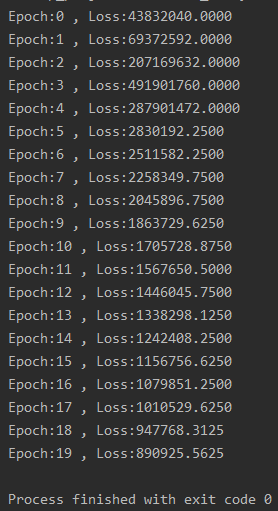## 3、使用自动梯度和自定义函数搭建简易神经网络模型

import torch

#构建神经网络模型
class Model(torch.nn.Module):
def __init__(self):
super(Model,self).__init__()
#super().__init__()
def forward(self,input,w1,w2):
x=torch.mm(input,w1)
x=torch.clamp(x,min=0)
x=torch.mm(x,w2)
return x
def backward(self):
pass

batch_n = 100 # 批量输入的数据量
hidden_layer = 100 # 通过隐藏层后输出的特征数
input_data = 1000 # 输入数据的特征个数
output_data = 10 # 最后输出的分类结果数
#初始化权重
epoch_n = 20  #训练的次数
learning_rate = 1e-6 #学习效率
model=Model() #对模型类进行调用
#模型训练和参数优化
for epoch in range(epoch_n):
y_pred = model(x,w1,w2) #完成对模型预测值的输出
loss = (y_pred - y).pow(2).sum()
print("Epoch:{} , Loss:{:.4f}".format(epoch, loss.data))
loss.backward()  #自动计算所有梯度
w2.grad.data.zero_()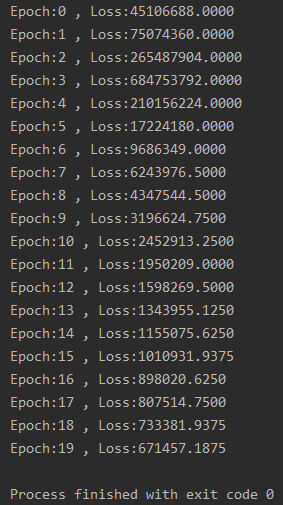（1）class Model(torch.nn.Module)

（3）.backward()函数

（4）.zero_()函数

# 三、torch.nn和torch.optim

torch.nn 包提供了很多与实现神经网络中的具体功能相关的类，这些类涵盖了深度神经网络模型在搭建和参数优化过程中的常用内容，比如神经网络中的卷积层、池化层、全连接层这类层次构造的方法、防止过拟合的参数归一化方法、Dropout 方法，还有激活函数部分的线性激活函数、非线性激活函数相关的方法等。

## 1、使用torch.nn搭建神经网络模型

# _*_coding:utf-8_*_
import torch

batch_n = 100  # 批量输入的数据量
input_data = 1000 # 输入数据的特征个数
hidden_layer = 100 # 通过隐藏层后输出的特征数
output_data = 10 # 最后输出的分类结果数

# 模型搭建
models = torch.nn.Sequential(
# 首先通过其完成从输入层到隐藏层的线性变换
torch.nn.Linear(input_data, hidden_layer),
# 经过激活函数
torch.nn.ReLU(),
# 最后完成从隐藏层到输出层的线性变换
torch.nn.Linear(hidden_layer, output_data)
)
epoch_n = 10000
learning_rate = 1e-4
loss_fn = torch.nn.MSELoss()  #均方误差损失函数
# 迭代优化
for epoch in range(epoch_n):
y_pred = models(x)
loss = loss_fn(y_pred, y)
if epoch % 1000 == 0:
print("Epoch:{},Loss:{:.4f}".format(epoch, loss.data))
loss.backward()
# 访问模型中的全部参数是通过对“models.parameters()”进行遍历完成的
# 对每个遍历的参数进行梯度更新
for param in models.parameters():
param.data -= param.grad.data * learning_rate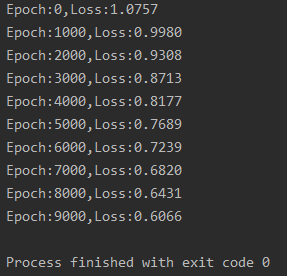（1）torch.nn.Sequential

torch.nn.Sequential 类是torch.nn中的一种序列容器，通过在容器中嵌套各种实现神经网络中具体功能相关的类，来完成对神经网络模型的搭建，最主要的是，参数会按照我们定义好的序列自动传递下去。我们可以将嵌套在容器中的各个部分看作各种不同的模块，这些模块可以自由组合。

import torch

batch_n = 100  # 批量输入的数据量
input_data = 1000 # 输入数据的特征个数
hidden_layer = 100 # 通过隐藏层后输出的特征数
output_data = 10 # 最后输出的分类结果数

#模型搭建
models = torch.nn.Sequential(
# 首先通过其完成从输入层到隐藏层的线性变换
torch.nn.Linear(input_data, hidden_layer),
# 经过激活函数
torch.nn.ReLU(),
# 最后完成从隐藏层到输出层的线性变换
torch.nn.Linear(hidden_layer, output_data))
print(models)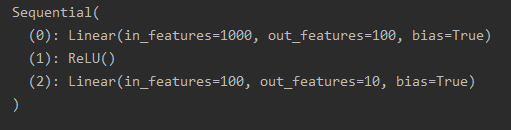import torch
from collections import OrderedDict

batch_n = 100  # 批量输入的数据量
input_data = 1000 # 输入数据的特征个数
hidden_layer = 100 # 通过隐藏层后输出的特征数
output_data = 10 # 最后输出的分类结果数

#模型搭建
models = torch.nn.Sequential(OrderedDict([
("Linel",torch.nn.Linear(input_data,hidden_layer)),
("ReLU1",torch.nn.ReLU()),
("Line2",torch.nn.Linear(hidden_layer,output_data))]))
print(models)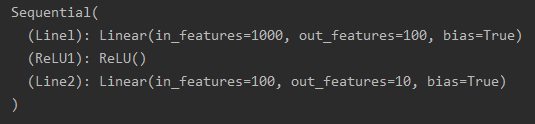（2）torch.nn.ReLU

torch.nn.ReLU 类属于非线性激活分类，在定义时默认不需要传入参数。在 torch.nn包中还有许多非线性激活函数类可供选择，比如PReLU、LeakyReLU、Tanh、Sigmoid、Softmax等。

（3）torch.nn.Linear

torch.nn.Linear 类用于定义模型的线性层，即完成前面提到的不同的层之间的线性变换。它接收的参数有三个，分别是输入特征数、输出特征数和是否使用偏置，设置是否使用偏置的参数是一个布尔值，默认为True，即使用偏置。

（4）torch.nn.MSELoss

torch.nn.MSELoss 类使用均方误差函数对损失值进行计算，在定义类的对象时不用传入任何参数，但在使用实例时需要输入两个维度一样的参数方可进行计算。

import torch

loss_f=torch.nn.MSELoss()
x=Variable(torch.randn(100,100))
y=Variable(torch.randn(100,100))
loss=loss_f(x,y)
print(loss)
tensor(2.0491)

## 2、使用torch.optim优化模型

# _*_coding:utf-8_*_
import torch

batch_n = 100  # 批量输入的数据量
input_data = 1000 # 输入数据的特征个数
hidden_layer = 100 # 通过隐藏层后输出的特征数
output_data = 10 # 最后输出的分类结果数

# 模型搭建
models = torch.nn.Sequential(
# 首先通过其完成从输入层到隐藏层的线性变换
torch.nn.Linear(input_data, hidden_layer),
# 经过激活函数
torch.nn.ReLU(),
# 最后完成从隐藏层到输出层的线性变换
torch.nn.Linear(hidden_layer, output_data)
)
epoch_n = 20
learning_rate = 1e-4
loss_fn = torch.nn.MSELoss()  # 均方误差损失函数

# 训练模型
for epoch in range(epoch_n):
y_pred = models(x)
loss = loss_fn(y_pred, y)
print("Epoch:{},Loss:{:.4f}".format(epoch, loss.data))
loss.backward()
optimzer.step() # 进行梯度更新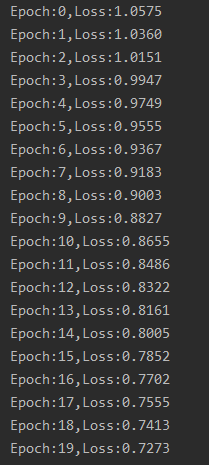optimzer.step 主要功能是使用计算得到的梯度值对各个节点的参数进行梯度更新。

1. PyTorch安装：PyTorch官网

2. Pytorch官方教程中文版

05-074251
11-2910万+02-226603
08-05524
11-057104
05-2336
06-063万+
08-08429
01-27288
06-098085